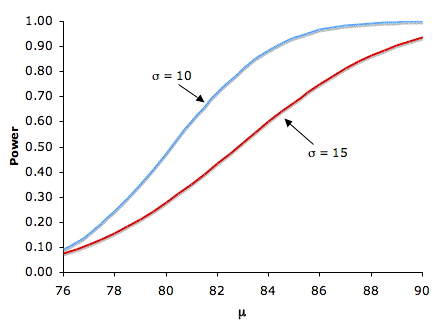# 13.5: Factors Affecting Power

•• David Lane
• Rice University
$$\newcommand{\vecs}{\overset { \rightharpoonup} {\mathbf{#1}} }$$ $$\newcommand{\vecd}{\overset{-\!-\!\rightharpoonup}{\vphantom{a}\smash {#1}}}$$$$\newcommand{\id}{\mathrm{id}}$$ $$\newcommand{\Span}{\mathrm{span}}$$ $$\newcommand{\kernel}{\mathrm{null}\,}$$ $$\newcommand{\range}{\mathrm{range}\,}$$ $$\newcommand{\RealPart}{\mathrm{Re}}$$ $$\newcommand{\ImaginaryPart}{\mathrm{Im}}$$ $$\newcommand{\Argument}{\mathrm{Arg}}$$ $$\newcommand{\norm}{\| #1 \|}$$ $$\newcommand{\inner}{\langle #1, #2 \rangle}$$ $$\newcommand{\Span}{\mathrm{span}}$$ $$\newcommand{\id}{\mathrm{id}}$$ $$\newcommand{\Span}{\mathrm{span}}$$ $$\newcommand{\kernel}{\mathrm{null}\,}$$ $$\newcommand{\range}{\mathrm{range}\,}$$ $$\newcommand{\RealPart}{\mathrm{Re}}$$ $$\newcommand{\ImaginaryPart}{\mathrm{Im}}$$ $$\newcommand{\Argument}{\mathrm{Arg}}$$ $$\newcommand{\norm}{\| #1 \|}$$ $$\newcommand{\inner}{\langle #1, #2 \rangle}$$ $$\newcommand{\Span}{\mathrm{span}}$$$$\newcommand{\AA}{\unicode[.8,0]{x212B}}$$

Learning Objectives

• State what the effect of each of the factors is

Several factors affect the power of a statistical test. Some of the factors are under the control of the experimenter, whereas others are not. The following example will be used to illustrate the various factors.

Suppose a math achievement test were known to be normally distributed with a mean of $$75$$ and a standard deviation of $$\sigma$$. A researcher is interested in whether a new method of teaching results in a higher mean. Assume that although the experimenter does not know it, the population mean $$\mu$$ for the new method is larger than $$75$$. The researcher plans to sample $$N$$ subjects and do a one-tailed test of whether the sample mean is significantly higher than $$75$$. In this section, we consider factors that affect the probability that the researcher will correctly reject the false null hypothesis that the population mean is $$75$$ or lower. In other words, factors that affect power.

## Sample Size

Figure $$\PageIndex{1}$$ shows that the larger the sample size, the higher the power. Since sample size is typically under an experimenter's control, increasing sample size is one way to increase power. However, it is sometimes difficult and/or expensive to use a large sample size.Figure $$\PageIndex{1}$$: The relationship between sample size and power for $$H_0: \mu = 75$$, real $$\mu = 80$$, one-tailed $$\alpha = 0.05$$, for $$\sigma 's$$ of $$10$$ and $$15$$

## Standard Deviation

Figure $$\PageIndex{1}$$ also shows that power is higher when the standard deviation is small than when it is large. For all values of $$N$$, power is higher for the standard deviation of $$10$$ than for the standard deviation of $$15$$ (except, of course, when $$N = 0$$). Experimenters can sometimes control the standard deviation by sampling from a homogeneous population of subjects, by reducing random measurement error, and/or by making sure the experimental procedures are applied very consistently.

## Difference between Hypothesized and True Mean

Naturally, the larger the effect size, the more likely it is that an experiment would find a significant effect. Figure $$\PageIndex{2}$$ shows the effect of increasing the difference between the mean specified by the null hypothesis ($$75$$) and the population mean $$\mu$$ for standard deviations of $$10$$ and $$15$$.Figure $$\PageIndex{2}$$: The relationship between $$\mu$$ and power for $$H_0: \mu = 75$$, one-tailed $$\alpha = 0.05$$, for $$σ's$$ of $$10$$ and $$15$$

## Significance Level

There is a trade-off between the significance level and power: the more stringent (lower) the significance level, the lower the power. Figure $$\PageIndex{3}$$ shows that power is lower for the $$0.01$$ level than it is for the $$0.05$$ level. Naturally, the stronger the evidence needed to reject the null hypothesis, the lower the chance that the null hypothesis will be rejected.Figure $$\PageIndex{3}$$: The relationship between significance level and power with one-tailed tests: $$μ = 75$$, real $$μ = 80$$, and $$σ = 10$$

## One- versus Two-Tailed Tests

Power is higher with a one-tailed test than with a two-tailed test as long as the hypothesized direction is correct. A one-tailed test at the $$0.05$$ level has the same power as a two-tailed test at the $$0.10$$ level. A one-tailed test, in effect, raises the significance level.

This page titled 13.5: Factors Affecting Power is shared under a Public Domain license and was authored, remixed, and/or curated by David Lane via source content that was edited to the style and standards of the LibreTexts platform; a detailed edit history is available upon request.